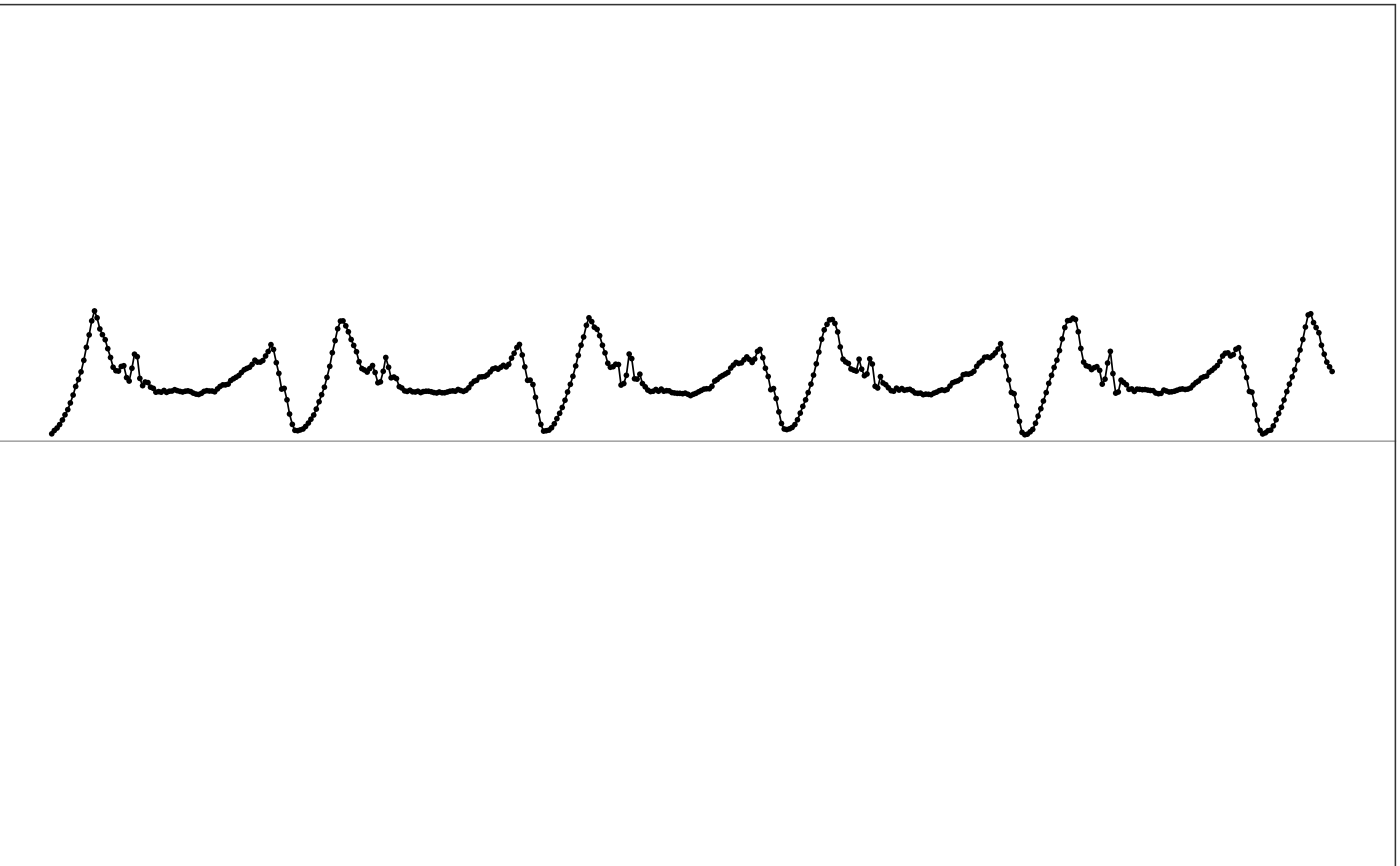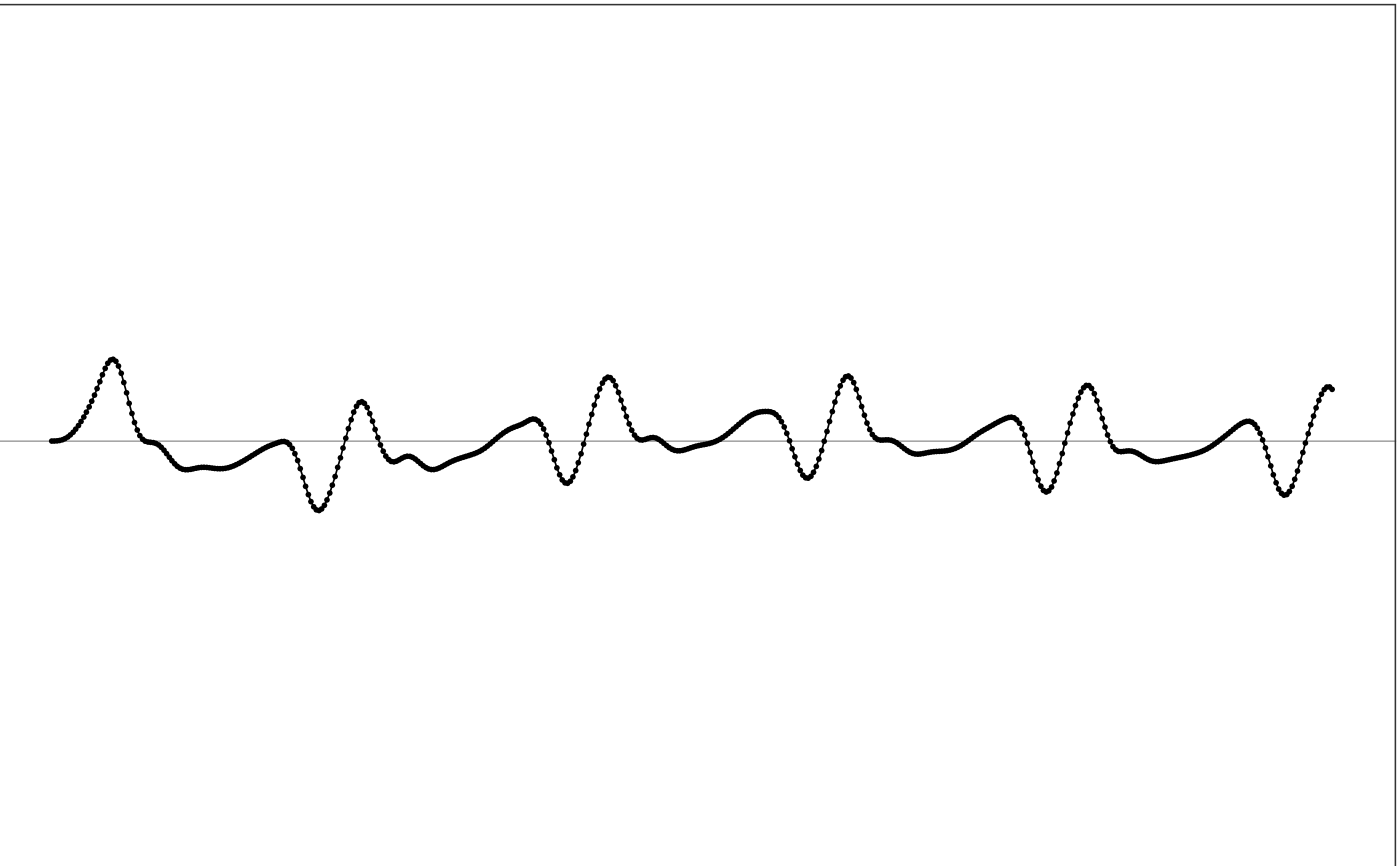iir function takes a multi-channel signal and applies an IIR filter to the signal.

iir(df, sr, cutoff_freq, order = 4, type = "high", filter_type = "butter")

## Arguments

df dataframe. The input multi-channel signal. The first column is timestamps in POSXlct format. The rest columns are signal values. number. Sampling rate in Hz of the input signal. number or numerical vector. The cutoff frequencies in Hz. If the IIR filter is a bandpass or bandstop filter, it will be a 2-element numerical vector specifying the low and high end cutoff frequencies c(low, high). number. The order of the filter. Default is 4. string. Filtering type, one of "low" for a low-pass filter, "high" for a high-pass filter, "stop" for a stop-band (band-reject) filter, or "pass" for a pass-band filter. string. IIR filter type, one of "butter" for butterworth filter, "chebyI" for Chebyshev Type I filter, or "ellip" for Elliptic filter.

## Value

dataframe. Filtered signal.

## Details

This function filters the input multi-channel signal by applying an IIR filter. See wiki for the explanation of the filter. The implementations of IIR filters can be found in butter, cheby1, and ellip.

For Chebyshev Type I, Type II and Elliptic filter, the passband ripple is fixed to be 0.05 dB. For Elliptic filter, the stopband ripple is fixed to be -50dB.

## How is it used in MIMS-unit algorithm?

This function has been used as the main filtering method in MIMS-unit algorithm. Specifically, it uses a 0.5 - 5 Hz bandpass butterworth filter during filtering.

## See also

Other filtering functions: bandlimited_interp(), bessel(), remove_average()

## Examples

  # Use sample data
df = sample_raw_accel_data

# View input
illustrate_signal(df, plot_maxed_out_line = FALSE)# Apply filtering that uses the same setting as in MIMSunit algorithm
output = iir(df, sr=80, cutoff_freq=c(0.2, 5), type='pass')

# View output
illustrate_signal(output, plot_maxed_out_line = FALSE)